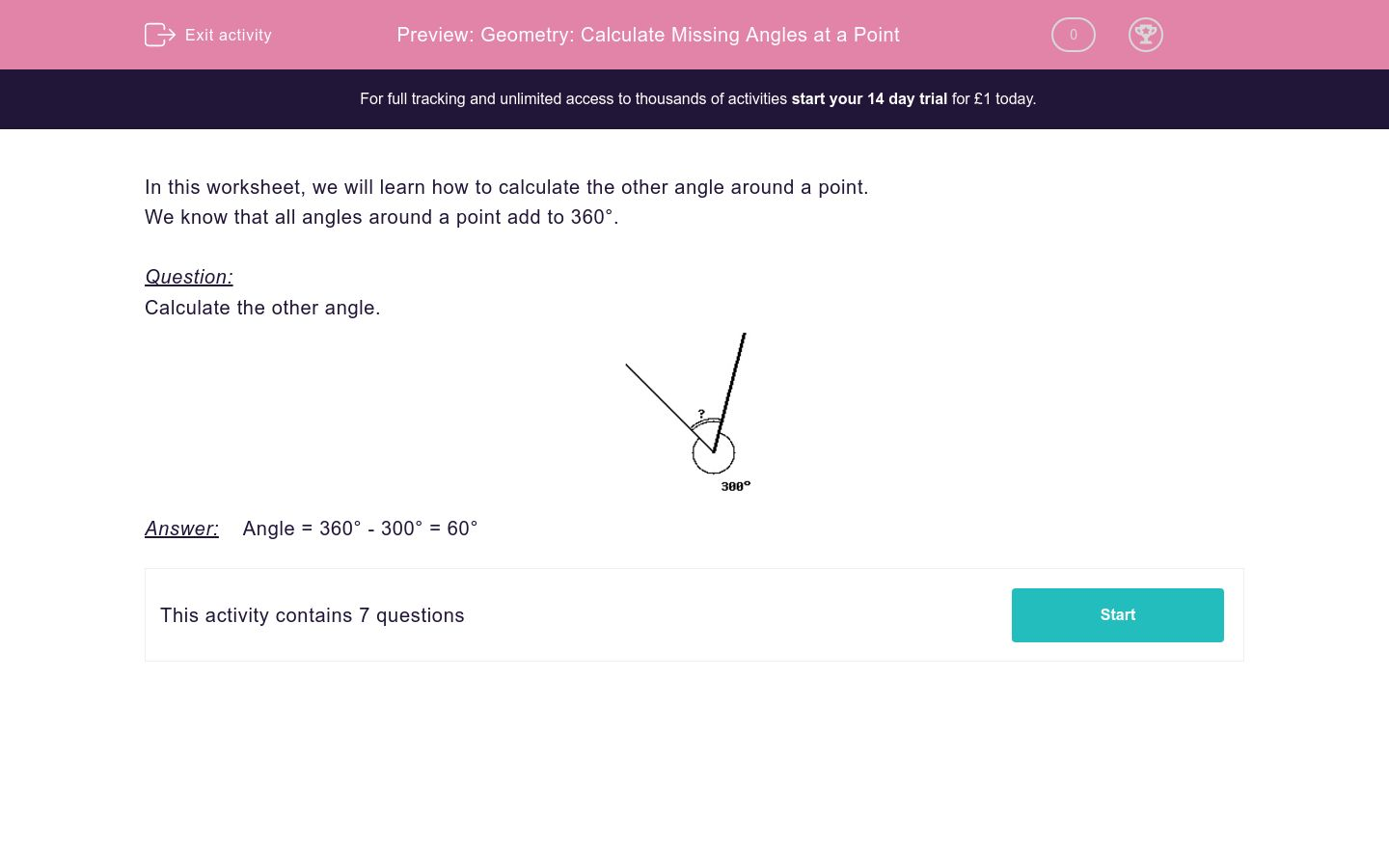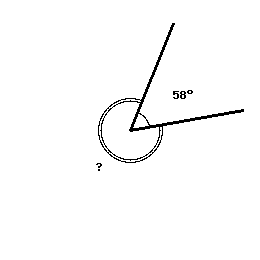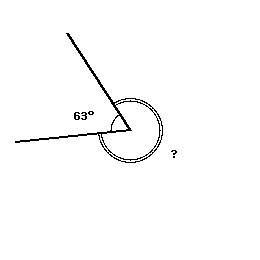# Geometry: Calculate Missing Angles at a Point

In this worksheet, students examine and calculate angles at the point where one whole turn is 360 degrees.Key stage:  KS 2

Curriculum topic:   Maths and Numerical Reasoning

Curriculum subtopic:   2D Shapes: Circles, Angles and Bearings

Difficulty level:### QUESTION 1 of 10

In this worksheet, we will learn how to calculate the other angle around a point.

We know that all angles around a point add to 360°.

Question:

Calculate the other angle.Answer:    Angle = 360° - 300° = 60°

Calculate the other angleCalculate the other angleCalculate the other angleCalculate the other angleCalculate the other angleCalculate the other angleCalculate the other angle• Question 1

Calculate the other angle152
EDDIE SAYS
Remember, the angles around a point add up to 360.
So, 360 - 208 = 152
• Question 2

Calculate the other angle302
EDDIE SAYS
360 - 58 = 302
• Question 3

Calculate the other angle200
EDDIE SAYS
360 - 160 = 200
• Question 4

Calculate the other angle313
EDDIE SAYS
360 - 47 = 313
• Question 5

Calculate the other angle154
EDDIE SAYS
360 - 206 = 154
• Question 6

Calculate the other angle285
EDDIE SAYS
360 - 75 = 285
• Question 7

Calculate the other angle297
EDDIE SAYS
360 - 63 = 297
---- OR ----

Sign up for a £1 trial so you can track and measure your child's progress on this activity.

### What is EdPlace?

We're your National Curriculum aligned online education content provider helping each child succeed in English, maths and science from year 1 to GCSE. With an EdPlace account you’ll be able to track and measure progress, helping each child achieve their best. We build confidence and attainment by personalising each child’s learning at a level that suits them.

Get started